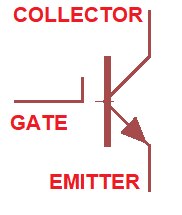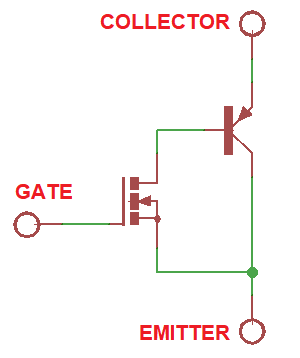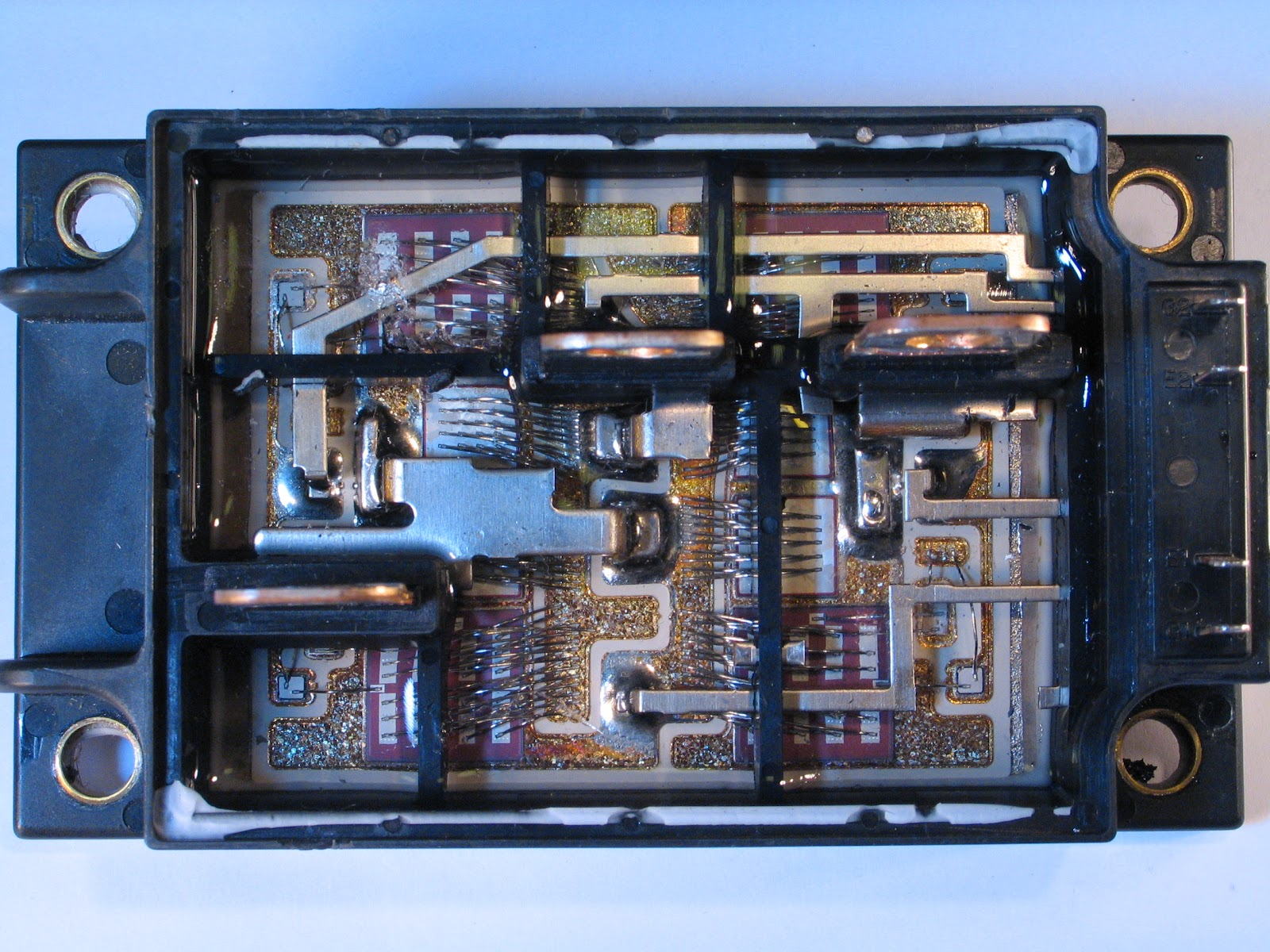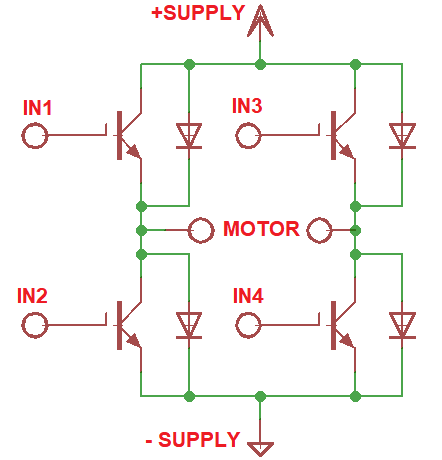Home / Circuits / IGBT Transistor Basics

# IGBT Transistor Basics

The IGBT or Insulated Gate Bipolar Transistor is a type of transistor. It’s a high-current and high-voltage device and is a thyristor. IGBT combines both the good traits of a Metal Oxide Field Effect Transistor (MOSFET) and a Bipolar Junction Transistor (BJT).

### Introduction

The IGBT’s schematic symbol is shown below:The likeness of the IGBT’s symbol to the BJT is intentional. Like the BJT, the IGBT’s output is current from the collector to the emitter. However, the insulated gate of this device means the input current at the gate is almost zero. A very small current is proportional to a very high impedance. Thus, loading does not affect the driving circuit connected to the gate of this transistor. The insulated-gate bipolar transistor is also superior to BJT (in some applications) in terms of switching speed.

Since the IGBT is a voltage-controlled device, it’s similar to the MOSFET. Its primary advantage over MOSFETs is its capability of handling over 200 V of collector-to-emitter voltage. Such transistor also have smaller saturation voltage than MOSFET.

### Operation

An equivalent circuit below best describes the operation of a IGBT:As seen, a MOSFET is on the input side of the insulated-gate bipolar transistor while a BJT is at the output side. Current will flow from collector to emitter when enough voltage is applied to the gate.

### Applications

IGBTs often come as modules. Such modules are, in-fact, solid-state relays. IGBT applications include motor drivers, induction heaters and power supplies.

An IGBT module looks like this:Below is an example schematic diagram of a motor driver circuit:Notice that the schematic diagram above is just like an H-bridge but only using insulated-gate bipolar transistors. The advantage of using this IGBT H-bridge is higher operating voltage and current.

## Voltage Divider Calculator

Introduction to Voltage Dividers Resistors are in series when the same current flows through them. …